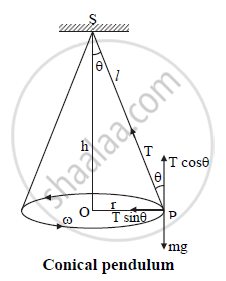# Draw a Neat Labelled Diagram of Conical Pendulum. State the Expression for Its Periodic Time in Terms of Length. - Physics

Sum

Draw a neat labelled diagram of conical pendulum. State the expression for its periodic time in terms of length.

#### SolutionWhere, S: rigid support

T : tension in the string

l : length of string

h : height of support from bob

v : velocity of bob

r : radius of horizontal circle

θ: semi-vertical angle

mg : weight of bob

i) Consider a bob of mass m tied to one
end of a string of length ‘l’ and other
the end is fixed to a rigid support.

ii)  Let the bob be displaced from its mean
position and whirled around a
horizontal circle of radius ‘r’ with
constant angular velocity ω, then the
bob performs U.C.M.

iii) During the motion, a string is inclined to
the vertical at an angle θ as shown in
the above figure.

iv)  In the displaced position P, there are two
forces acting on the bob.
a. The weight mg acting vertically
downwards.
b. The tension T acting upward
along the string.

v) The tension (T) acting in the string can
be resolved into two components:
a. T cos θ acting vertically upwards.
b. T sin θ acting horizontally towards
centre of the circle.

vi) Vertical component T cos θ balances the
weight and horizontal component T sin θ
provides the necessary centripetal force.

∴ T cos θ = mg    . ........(1)

T sin θ = "mv"^2/"r" = "mr"omega^2  ....(2)

vii)  Dividing equation (2) by (1),

tan θ = "v"^2/"rg"     ......(3)

Therefore, the angle made by the string with the vertical is  θ = tan^-1 ("v"^2/"rg")

Also, from equation (3),

v2 = rg tan θ

∴ v = sqrt ("rg" tan theta)

The period, T = (2pi"r")/"v" = (2pi"r")/sqrt("rg" "tan" theta) = 2pi sqrt ("r"/("g tan" theta))

It can be seen that r - = l sin θ

T = 2pi sqrt (("l sin" theta)/("g tan" theta)) = 2pi sqrt (("l cos" theta)/"g")

The period of a conical pendulum is 2pi sqrt (("l cos" theta)/"g")

Concept: Uniform Circular Motion
Is there an error in this question or solution?